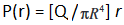# Let  be the charge density distribution for a solid sphere of radius R and total charge Q. For a point ‘p’ inside the sphere at...

Letbe the charge density distribution for a solid sphere of radius R and total charge Q. For a point ‘p’ inside the sphere at distance r1 from the centre of the sphere, what will be the magnitude of electric field?

Anonymous User Physics Electrostatic 06 May, 2020 54 views